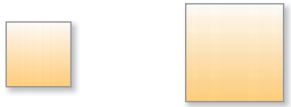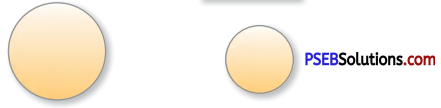# PSEB 4th Class Maths Solutions Chapter 8 Perimeter and Area Ex 8.2

Punjab State Board PSEB 4th Class Maths Book Solutions Chapter 8 Perimeter and Area Ex 8.2 Textbook Exercise Questions and Answers.

## PSEB Solutions for Class 4 Maths Chapter 8 Perimeter and Area Ex 8.2

Which of the following figures have covered more surface. Tick the figure which (✓) has greater area.

(a)Solution:
First Figure

(b)Solution:
Second Figure(c)Solution:
First Figure